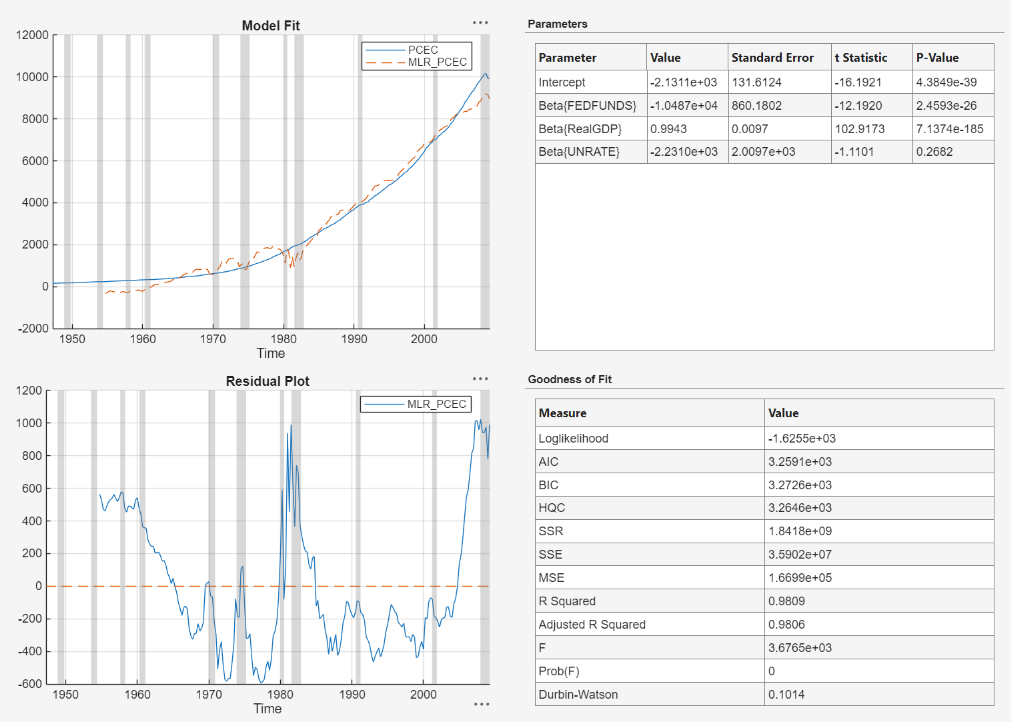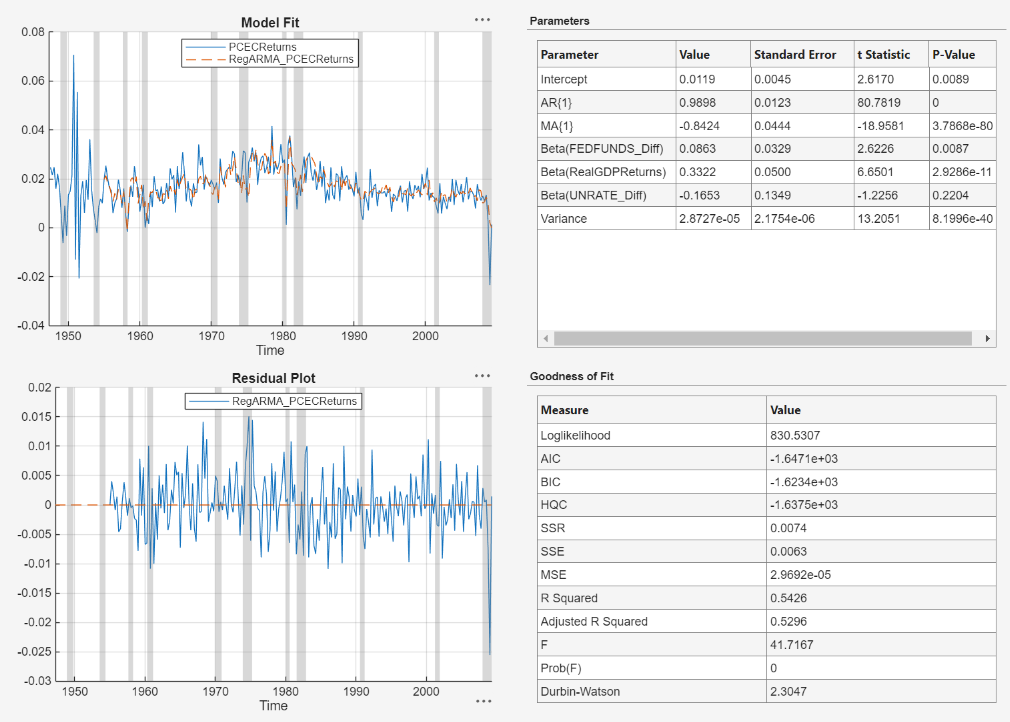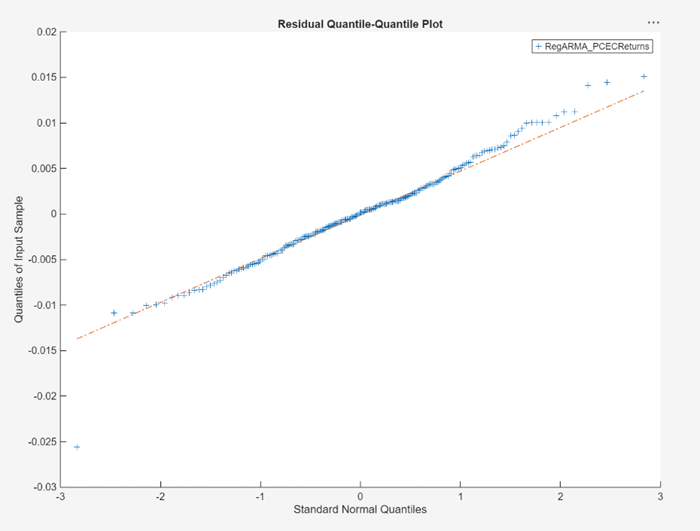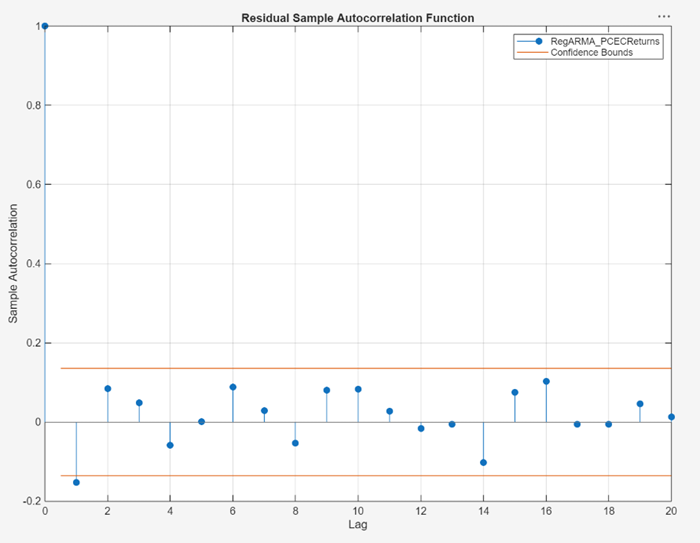Documentation

## Estimate Regression Model with ARMA Errors Using Econometric Modeler App

This example shows how to specify and estimate a regression model with ARMA errors using the Econometric Modeler app. The data set, which is stored in `Data_USEconModel.mat`, contains the US personal consumption expenditures measured quarterly, among other series.

Consider modeling the US personal consumption expenditures (`PCEC`, in \$ billions) as a linear function of the effective federal funds rate (`FEDFUNDS`), unemployment rate (`UNRATE`), and real gross domestic product (`GDP`, in \$ billions with respect to the year 2000).

### Import Data into Econometric Modeler

At the command line, load the `Data_USEconModel.mat` data set.

`load Data_USEconModel`

Convert the federal funds and unemployment rates from percents to decimals.

```DataTable.UNRATE = 0.01*DataTable.UNRATE; DataTable.FEDFUNDS = 0.01*DataTable.FEDFUNDS; ```

Convert the nominal GDP to real GDP by dividing all values by the GDP deflator (`GDPDEF`) and scaling the result by 100. Create a column in `DataTable` for the real GDP series.

```DataTable.RealGDP = 100*DataTable.GDP./DataTable.GDPDEF; ```

At the command line, open the Econometric Modeler app.

`econometricModeler`

Alternatively, open the app from the apps gallery (see Econometric Modeler).

Import `DataTable` into the app:

1. On the Econometric Modeler tab, in the Import section, click.

2. In the Import Data dialog box, in the Import? column, select the check box for the `DataTable` variable.

3. Click .

All time series variables in `DataTable` appear in the Data Browser, and a time series plot of the series appears in the Time Series Plot(COE) figure window.

### Plot the Series

Plot the `PCEC`, `RealGDP`, `FEDFUNDS`, and `UNRATE` series on separate plots.

1. In the Data Browser, double-click `PCEC`.

2. Repeat step 1 for `RealGDP`, `FEDFUNDS`, and `UNRATE`.

3. In the right pane, drag the Time Series Plot(PCEC) figure window to the top so that it occupies the first two quadrants.

4. Drag the Time Series Plot(RealGDP) figure window to the first quadrant.

5. Drag the Time Series Plot(UNRATE) figure window to the third quadrant.The `PCEC` and `RealGDP` series appear to have an exponential trend. The `UNRATE` and `FEDFUNDS` series appear to have a stochastic trend.

Right-click the tab for any figure window, then select Close All to close all the figure windows.

### Assess Collinearity Among Series

Check whether the series are collinear by performing Belsley collinearity diagnostics.

1. In the Data Browser, select `PCEC`. Then, press Ctrland click to select `RealGDP`, `FEDFUNDS`, and `UNRATE`.

2. On the Econometric Modeler tab, in the Tests section, click > Belsley Collinearity Diagnostics.

The Belsley collinearity diagnostics results appear in the Collinearity(FEDFUNDS) document.All condition indices are below the default condition-index tolerance, which is 30. The time series do not appear to be collinear.

### Specify and Estimate Linear Model

Specify a linear model in which `PCEC` is the response and `RealGDP`, `FEDFUNDS`, and `UNRATE` are predictors.

1. In the Data Browser, select `PCEC`.

2. Click the Econometric Modeler tab. Then, in the Models section, click the arrow to display the models gallery.

3. In the models gallery, in the Regression Models section, click MLR.

4. In the MLR Model Parameters dialog box, in the Predictors section, select the Include? check box for the `FEDFUNDS`, `RealGDP`, and `UNRATE` time series.5. Click .

The model variable `MLR_PCEC` appears in the Models section of the Data Browser, and its estimation summary appears in the Model Summary(MLR_PCEC) document.In the Model Summary(MLR_PCEC) figure window, the residual plot suggests that the standard linear model assumption of uncorrelated errors is violated. The residuals appear autocorrelated, nonstationary, and possibly heteroscedastic.

### Stabilize Variables

To stabilize the residuals, stabilize the response and predictor series by converting the `PCEC` and `RealGDP` prices to returns, and by applying the first difference to `FEDFUNDS` and `UNRATE`.

Convert `PCEC` and `RealGDP` prices to returns:

1. In the Data Browser, select the `PCEC` time series.

2. On the Econometric Modeler tab, in the Transforms section, click .

In the Data Browser, a variable representing the logged `PCEC` prices (`PCECLog`) appears.

3. In the Data Browser, select `PCECLog`.

4. On the Econometric Modeler tab, in the Transforms section, click .

In the Data Browser, a variable representing the first differences of the logged `PCEC` prices (`PCECLogDiff`) appears.

5. Rename the `PCECLogDiff` variable to `PCECReturns`.

1. In the Data Browser, right-click `PCECLogDiff`.

2. In the context menu, select Rename.

3. Enter `PCECReturns`.

6. Repeat steps 1 through 5, but select the `RealGDP` time series instead. Rename the real GDP returns to `RealGDPReturns`.

Apply the first difference to `FEDFUNDS` and `UNRATE`:

1. In the Data Browser, select the `FEDFUNDS` time series.

2. On the Econometric Modeler tab, in the Transforms section, click .

In the Data Browser, a variable representing the first difference of `FEDFUNDS` (`FEDFUNDSDiff`) appears.

3. Repeat steps 1 and 2, but select the `UNRATE` time series instead.

4. Close all figure windows and documents.

### Respecify and Estimate Linear Model

Respecify the linear model, but use the stabilized series instead.

1. In the Data Browser, select `PCECReturns`.

2. On the Econometric Modeler tab, in the Models section, click the arrow to display the models gallery.

3. In the models gallery, in the Regression Models section, click MLR.

4. In the MLR Model Parameters dialog box, in the Predictors section, select the Include? check box for the `FEDFUNDSDiff`, `RealGDPReturns`, and `UNRATEDiff` time series.

5. Click .

The model variable `MLR_PCECReturns` appears in the Models section of the Data Browser, and its estimation summary appears in the Model Summary(MLR_PCECReturns) document.The residual plot suggests that the residuals are autocorrelated.

### Check Goodness of Fit of Linear Model

Assess whether the residuals are normally distributed and autocorrelated by generating quantile-quantile and ACF plots.

Create a quantile-quantile plot of the `MLR_PCECReturns` model residuals:

1. In the Data Browser, select the `MLR_PCECReturns` model.

2. On the Econometric Modeler tab, in the Diagnostics section, click > Residual Q-Q Plot.The residuals are skewed to the right.

Plot the ACF of the residuals:

1. In the Data Browser, select the `MLR_PCECReturns` model.

2. On the Econometric Modeler tab, in the Diagnostics section, click > Autocorrelation Function.

3. On the ACF tab, set Number of Lags to `40`.The plot shows autocorrelation in the first 34 lags.

### Specify and Estimate Regression Model with ARMA Errors

Attempt to remedy the autocorrelation in the residuals by specifying a regression model with ARMA(1,1) errors for `PCECReturns`.

1. In the Data Browser, select `PCECReturns`.

2. Click the Econometric Modeler tab. Then, in the Models section, click the arrow to display the models gallery.

3. In the models gallery, in the Regression Models section, click RegARMA.

4. In the regARMA Model Parameters dialog box:

1. In the Lag Order tab:

1. Set Autoregressive Order to `1`.

2. Set Moving Average Order to `1`.

2. In the Predictors section, select the Include? check box for the `FEDFUNDSDiff`, `RealGDPReturns`, and `UNRATEDiff` time series.3. Click .

The model variable `RegARMA_PCECReturns` appears in the Models section of the Data Browser, and its estimation summary appears in the Model Summary(RegARMA_PCECReturns) document.The t statistics suggest that all coefficients are significant, except for the coefficient of `UNRATEDiff`. The residuals appear to fluctuate around y = 0 without autocorrelation.

### Check Goodness of Fit of ARMA Error Model

Assess whether the residuals of the `RegARMA_PCECReturns` model are normally distributed and autocorrelated by generating quantile-quantile and ACF plots.

Create a quantile-quantile plot of the `RegARMA_PCECReturns` model residuals:

1. In the Data Browser, select the `RegARMA_PCECReturns` model.

2. On the Econometric Modeler tab, in the Diagnostics section, click > Residual Q-Q Plot.The residuals appear approximately normally distributed.

Plot the ACF of the residuals:

1. In the Data Browser, select the `RegARMA_PCECReturns` model.

2. On the Econometric Modeler tab, in the Diagnostics section, click > Autocorrelation Function.The first autocorrelation lag is significant.

From here, you can estimate multiple models that differ by the number of autoregressive and moving average polynomial orders in the ARMA error model. Then, choose the model with the lowest fit statistic. Or, you can check the predictive performance of the models by comparing forecasts to out-of-sample data.